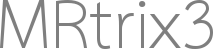# 2x2 Mixed design

Dear fellow MRtrix community members,

First a hugh compliment for the clarity of the documentation on performing fixel based morphometry!
I’ve been working through the documentation but I have a question about the last part: the CFE stats.
I have a 2x2 mixed design (2 groups, 2 time points) for which I need to prepare a design matrix. I read in this post: Design matrix for fixelcfestats
that I can follow the same rules as with the GLM specification in FSL. Nevertheless, the fsl documentation states that GLM, and randomise in particular, is ill equipped to handle a repeated measures design such as mine (https://fsl.fmrib.ox.ac.uk/fsl/fslwiki/GLM#ANOVA:2-groups.2C_2-levels_per_subject.282-way_Mixed_Effect_ANOVA.29).
Does this also apply to CFE stats or can I still (in some way or form) perform this analyses?

best wishes, Chris

Welcome Chris!

While the GLM can’t handle such an experiment in the mathematically “purest” sense, the non-idealities introduced by using the pragmatic solution shouldn’t really cause issues except for the most unusual of circumstances.

Assuming that you have two time points for all subjects, and the duration between your two time points is reasonably equivalent between subjects, the solution is to pre-calculate a fixel data file for each subject representing the difference between the two time points (or personally I’d favour pre-calculating the rate of change per unit time for each subject). You then have one input image per subject for statistical inference. If your primary hypothesis is e.g. that the rate of change of your quantitative metric over time is different between the two groups, then you could have one design matrix column calculating the mean rate of change in one group, a separate column for the second group, and a row in your contrast matrix subtracting one of these rates of change from the other, with the null hypothesis being that the value of that subtraction is zero.

It means that the distribution of the values provided as input to `fixelcfestats` is different to that of the original quantitative metric, and the variance of those derivative measures is not independent of the duration between acquisitions; but any loss of precision from non-normality is likely overwhelmed by the combination of intrinsic data variance and heuristic statistical enhancement anyway.

If there’s some other detail of your experiment that makes this approach unsuitable, I’d probably need access to those details in order to be able to better advise.

Cheers
Rob

Hi Rob,

thanks for your reply! My experimental design involves a randomized controlled trial comparing two conditions (experimental and control) with pre-post imaging. The duration between the two time points is therefore pretty equal between the groups. My hypothesis is that the change in one condition is higher than in the other.

When you say “pre-calculating the rate of change per unit time for each subject” do you mean calculating the difference between the subject-specific fixel maps and divide by the number of days between them?

Another thought that occured to me the other day is that there are studies that show that adjusting for the subject-specific baseline values in an ANCOVA has more power to detect effects than a repeated measures design. That would require the baseline fixel map to be used as a covariate. This is something I think you can do in FSL by adding a voxel-dependent EV. Is such an option also available in MRtrix3?